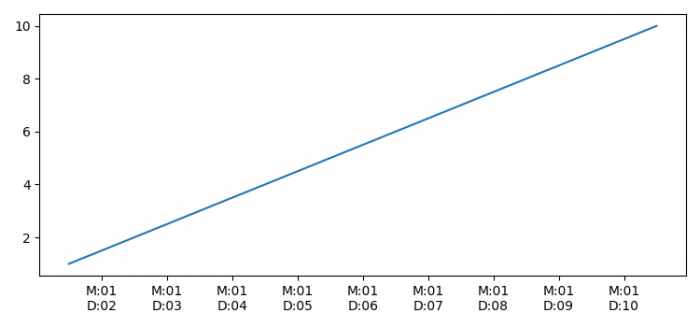# How to extract only the month and day from a datetime object in Python?

To extract only the month and day from a datetime object in Python, we can use the DateFormatter() class.

## steps

• Set the figure size and adjust the padding between and around the subplots.

• Make a dataframe, df, of two-dimensional, size-mutable, potentially heterogeneous tabular data.

• Create a figure and a set of subplots.

• Plot the dataframe using plot() method.

• Set the axis formatter, extract month and day.

• To display the figure, use show() method.

## Example

import numpy as np
import pandas as pd
from matplotlib import pyplot as plt, dates

plt.rcParams["figure.figsize"] = [7.50, 3.50]
plt.rcParams["figure.autolayout"] = True

df = pd.DataFrame(dict(time=list(pd.date_range("2021-01-01 12:00:00", periods=10)), speed=np.linspace(1, 10, 10)))

fig, ax = plt.subplots()

ax.plot(df.time, df.speed)
ax.xaxis.set_major_formatter(dates.DateFormatter('M:%m\nD:%d'))

plt.show()

## Output